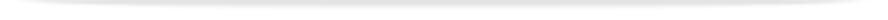units

MTH2032

Faculty of Science

# Undergraduate - UnitMTH2032 - Differential equations with modelling

This unit entry is for students who completed this unit in 2015 only. For students planning to study the unit, please refer to the unit indexes in the the current edition of the Handbook. If you have any queries contact the managing faculty for your course or area of study.print version

## 6 points, SCA Band 2, 0.125 EFTSL

Refer to the specific census and withdrawal dates for the semester(s) in which this unit is offered.

 Level Undergraduate Faculty Faculty of Science Organisational Unit School of Mathematical Sciences Offered Clayton Second semester 2015 (Day) Coordinator(s) Dr Jerome Droniou

### Synopsis

The study of Models of physical problems particularly heat conduction and oscillations, using computer simulation, laboratory experiments and mathematical analysis. Computer algorithms and mathematical techniques for ordinary differential equations using Euler and Predictor-Corrector methods with exact solutions found using separation of variables, integrating factors and substitution methods. The partial differential equations for material transport, heat conduction and wave motion are derived using physical models and solved using separation of variables and computational algorithms. Applications are to wave motion and heat conduction in a variety of practical situations.

### Outcomes

On completion of this unit students will be able to:

1. Describe various classes of ordinary and partial differential equations and the physical systems to which they apply;

1. Identify the differential equations that describe various physical process including those for simple harmonic motion, diffusion, wave propagation and potential-driven systems;

1. Describe the essential properties of the solutions of those differential equations;

1. Construct solutions of differential equations using analytic, computational and graphical methods;

1. Use computer algebra packages to assist in solving and interpreting the solution of differential equations;

1. Appreciate the role that differential equations and their solutions play in the scientific process, in particular their use as a tool to model physical systems and allow predictions to be made and tested.

### Assessment

Examination (3 hours): 60%
Assignments: 15%
Mid-semester test: 15%
Quizzes: 10%

Three 1-hour lectures and one 1.5 hour workshop per week